# Test: Areas Of Similar Triangles

## 10 Questions MCQ Test Mathematics (Maths) Class 10 | Test: Areas Of Similar Triangles

Description
This mock test of Test: Areas Of Similar Triangles for Class 10 helps you for every Class 10 entrance exam. This contains 10 Multiple Choice Questions for Class 10 Test: Areas Of Similar Triangles (mcq) to study with solutions a complete question bank. The solved questions answers in this Test: Areas Of Similar Triangles quiz give you a good mix of easy questions and tough questions. Class 10 students definitely take this Test: Areas Of Similar Triangles exercise for a better result in the exam. You can find other Test: Areas Of Similar Triangles extra questions, long questions & short questions for Class 10 on EduRev as well by searching above.
QUESTION: 1

### In similar triangles ABC and DEF, AG and DH are the medians. What is the value of AG/DH  given that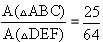​

Solution:

If two triangles are similar, then the ratio of the area of both triangles is proportional to the square of the ratio of their corresponding sides. So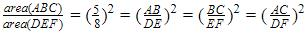Also
ΔABGand ΔDEFare also similar triangles , since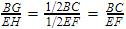, so by SAS criterion they are similar.And we know ratios of sides is ⅝ , so AG/DH=5/8

QUESTION: 2

Solution:
QUESTION: 3

### Triangle ABC is similar to triangle DEF and their areas are 64 cm2 and 121 cm2 respectively. If EF = 15.4 cm, then BC = ?​

Solution:

By Similar Triangle Theorem we get,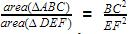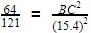By taking square roots of both sides,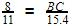BC=11.2

QUESTION: 4

In ΔDEF and ΔPQR, ∠D = 30°, ∠P = 30°, ∠E = 50°, ∠Q = 50° DE = 7 cm, PQ = 13cm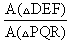= ?​

Solution:
QUESTION: 5

The ratio of the areas of two similar triangles is equal to the:​

Solution:
QUESTION: 6

Triangle ABC is similar to triangle DEF and their areas are respectively 64 cm2and 121 cm2. If EF = 15.4 cm, then BC =​

Solution:
QUESTION: 7

Two similar triangles ABC and DEF are such that, AB = 5cm, DE = 12cm, then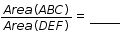Solution:
QUESTION: 8

Two similar triangles ABC and EDF are such that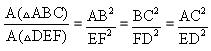Which of the following is true?​

Solution:
QUESTION: 9

Sides of two similar triangles are in the ratio 4: 9. What is the ratio of the area of these triangles?​

Solution:
QUESTION: 10

Two similar triangles ABC and DEF are such that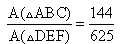.  What is the ratio of their corresponding sides?​

Solution: